Next: Runge-Kutta methods Up: Integration of ODEs Previous: Numerical errors

## Numerical instabilities

Consider the following example. Suppose that our o.d.e. is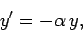(14)

where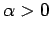, subject to the boundary condition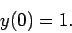(15)

Of course, we can solve this problem analytically to give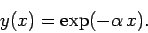(16)

Note that the solution is a monotonically decreasing function of. We can also solve this problem numerically using Euler's method. Appropriate grid-points are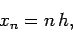(17)

where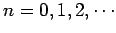. Euler's method yields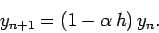(18)

Note one curious fact. If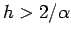then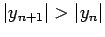. In other words, if the step-length is made too large then the numerical solution becomes an oscillatory function ofof monotonically increasing amplitude: i.e., the numerical solution diverges from the actual solution. This type of catastrophic failure of a numerical integration scheme is called a numerical instability. All simple integration schemes become unstable if the step-length is made sufficiently large.Next: Runge-Kutta methods Up: Integration of ODEs Previous: Numerical errors
Richard Fitzpatrick 2006-03-29